# Approximate differentiability

(diff) ← Older revision | Latest revision (diff) | Newer revision → (diff)

A generalization of the concept of differentiability obtained by replacing the ordinary limit by an approximate limit. A real-valued function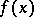of a real variable is called approximately differentiable at a point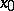if there exists a numbersuch thatThe magnitude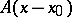is called the approximate differential ofat. A functionis approximately differentiable at a pointif and only if it has an approximate derivativeat this point. Approximate differentiability of real functions ofreal variables is defined in a similar manner. For example, for,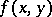is called approximately differentiable at a point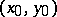if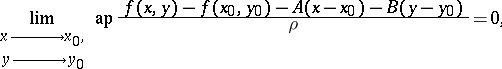whereandare certain given numbers and. The expression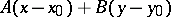is called the approximate differential ofat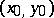.

Stepanov's theorem: A real-valued measurable functionon a setis approximately differentiable almost-everywhere on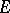if and only if it has finite approximate partial derivatives with respect toand toalmost-everywhere on; these partial derivatives almost-everywhere oncoincide with the coefficientsand, respectively, of the approximate differential.

The concept of approximate differentiability can also be extended to vector functions of one or more real variables.

How to Cite This Entry:
Approximate differentiability. Encyclopedia of Mathematics. URL: http://encyclopediaofmath.org/index.php?title=Approximate_differentiability&oldid=13557
This article was adapted from an original article by G.P. Tolstov (originator), which appeared in Encyclopedia of Mathematics - ISBN 1402006098. See original article